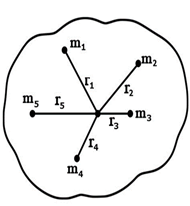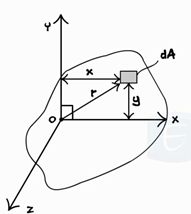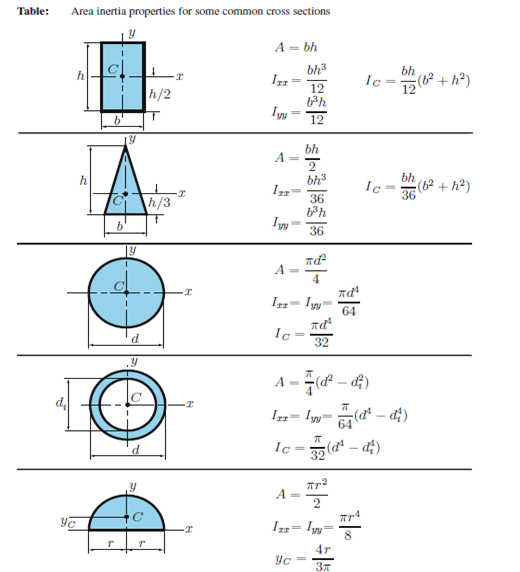# Perpendicular Axis Theorem

For understating what is perpendicular axis theorem, let’s take an object like a ball or a rotating disc which can rotate about its centre. You already know the moment of inertia of an object about its centre. But, After changing the point of rotation of this object, how do you find the Moment of Inertia? For understanding this, we have to know about Perpendicular axis theorem.

Now, before discussing the Perpendicular axis theorem, we will first see what moment of inertia is.

## Moment of Inertia

The property to resist angular acceleration is defined as the moment of inertia of an object. It is written as a summation of the products of the mass of each particle within the object, with the square of its distance from the axis of rotation.• Let’s consider an object which is having mass m.
• It consists of small particles which are having masses m1, m2, m3…….respectively.
• The perpendicular distance of each particle from the centre of mass is r1, r2, r3…… (as shown in the figure)

According to the definition of Moment of Inertia, the mass moment of Inertia for the whole object is:

I = m1r12 + m1r22 + m1r32 + …..

As we consider the mass of a body (m) to be concentrated at a point. That point is its centre of mass. If this mass m is situated at a perpendicular distance of r from the centre of mass then Moment of Inertia of the whole object is,

I = ∑ mr2

So to calculate the moment of Inertia, we use two important theorems. First one is Parallel Axis theorem and the second one is Perpendicular Axis theorem. In this article, we will emphasise only on perpendicular axis theorem. Let us understand what this concept is all about.

## What is Perpendicular Axis Theorem?

M.O.I of a 2-dimensional object about an axis passing perpendicularly from it is equal to the sum of the M.O.I of the object about 2 mutually perpendicular axes lying in the plane of the object.

According to the above definition of Perpendicular axis theorem can be written as,

IZZ = IXX + IYY

Also Read: How To Calculate Moment Of Inertia

### Proof of Perpendicular Axis Theorem

Let us assume there are three mutually perpendicular axes named as X, Y and Z. They are meeting at origin O.

Now consider the object lies in the XY plane having a small area dA. It is having y distance from X-axis and x distance from Y-axis. Its distance from the origin is r.Let IZ, IX and IY be moments of Inertia about the X, Y and Z axis respectively.

Moment of Inertia about Z-axis i.e.

IZ = ∫ r2.dA …………. (i)

Here, r2 = x2 + y2

Put this value in the above equation

IZZ = ∫ (x2 + y2) . dA

IZZ = ∫ x2.dA + y2.dA

IZZ = IXX + IYY

Hence proved.

## Difference Between Perpendicular Axis Theorem And Parallel Axis Theorem

 Parallel Axis Theorem Perpendicular Axis Theorem Moment of Inertia about any axis is equal to the sum of the Moments of Inertia about an axis parallel to this axis, passing through the Centre Of Mass (COM) and the product of the mass of the body with the square of the perpendicular distance between the axis in consideration and the COM axis parallel to it. M.O.I of a body about an axis passing perpendicularly from it is equal to the sum of the M.O.I of the body about 2 mutually perpendicular axes lying in the plane of the object. Io = IC + md2 Here, Io = M.O.I of shape about point O IC = M.O.I of shape about centroid ( C ) md2 = Added M.O.I due to the distance between O and C. IZZ = IXX + IYY This theorem is applicable for any object. There is no such restriction. This theorem applies only to the planar bodies that mean two-dimensional bodies.

## Application of Perpendicular Axis Theorem

• This theorem is most useful when considering a body which is symmetrical about two out of the three axes. If the value of the moment of Inertia about these two axes is known, then we can easily calculate the Moment of Inertia about the third axis by applying this theorem.
• For calculating M.O.I. of 3-dimensional objects such as cylinders, we can use this theorem. For this, break the cylinder into planner disks and then sum-up all the M.O.I. of the composite disks.

There is a table representing the Moment of Inertia of different bodies.